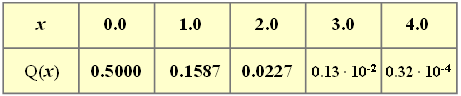# Exercise 3.11: Chebyshev's Inequality

(diff) ← Older revision | Latest revision (diff) | Newer revision → (diff)Values of the  "complementary Gaussian error function"

If nothing else is known about a random variable  $x$  than only

• the mean value  $m_x$,  and
• the standard deviation  $\sigma_x$,

so the  "Chebyshev's Inequality"  gives an upper bound on the probability that $x$  deviates by more than a value  $\varepsilon$  from its mean.  This bound is:

$${\rm Pr}(|x-m_x|\ge \varepsilon) \le {\sigma_x^{\rm 2}}/{\varepsilon^{\rm 2}}.$$

To explain:

• In the graph,  this upper bound is drawn in red.
• The green curve shows the actual probability for the uniform distribution.
• The blue points are for the exponential distribution.

From this plot it can be seen that the  "Chebyshev's Inequality"  is only a very rough bound.
It should be used only if really only the mean and the standard deviation are known from the random size.

Hints:

• On the right,  values of the complementary Gaussian error function  ${\rm Q}(x)$  are given.

### Questions

1

Which of the following statements are true?

 Conceivably,  a random variable with  ${\rm Pr}(|x -m_x | \ge 3\sigma_x) = 1/4$. "Chebyshev"  yields for  $\varepsilon < \sigma_x$  no information. ${\rm Pr}(|x -m_x | \ge \sigma_x)$  is identically zero for large  $\varepsilon$  if  $x$  is bounded.

2

It holds  $k = 1, \ 2, \ 3, \ 4$.  Give the excess probability  $p_k = {\rm Pr}(|x -m_x | \ge k \cdot \sigma_x)$  for the Gaussian distribution.  How large is  $p_3$?

 ${\rm Pr}(|x -m_x | \ge 3 \sigma_x) \ = \$ $\ \%$

3

What are the excess probabilities  $p_k$  for the  exponential distribution.  Here   $m_x = \sigma_x = 1/\lambda$.  What is  $p_3$?

 ${\rm Pr}(|x -m_x | \ge 3 \sigma_x) \ = \$ $\ \%$

### Solution

#### Solution

(1)  Correct are  the proposed solutions 2 and 3:

• The first statement is false.  Here,  Chebyshev's inequality provides the bound  $1/9$.
• For no distribution the probability considered here can be equal  $1/4$.
• For  $\varepsilon < \sigma_x$   ⇒   Chebyshev yields a probability greater  $1$.  This information is useless.
• The last statement is true.  For example,  with the uniform distribution:
$${\rm Pr}(| x- m_x | \ge \varepsilon)=\left\{ \begin{array}{*{4}{c}} 1-{\varepsilon}/{\varepsilon_{\rm 0}} & \rm for\hspace{0.1cm}{\it \varepsilon<\varepsilon_{\rm 0}=\sqrt{\rm 3}\cdot\sigma_x},\\\rm 0 & \rm else. \end{array} \right.$$

(2)  For the Gaussian distribution holds:

$$p_k={\rm Pr}(| x-m_x| \ge k\cdot\sigma_{x})=\rm 2\cdot \rm Q(\it k).$$
• This results in the following numerical values  $($in brackets:   bound according to Chebyshev$)$:
$$k= 1\text{:}\hspace{0.5cm} {\rm Pr}(|x-m_x| \ge \sigma_{x}) = 31.7 \% \hspace{0.3cm}(100 \%),$$
$$k= 2\text{:}\hspace{0.5cm} {\rm Pr}(|x-m_x| \ge 2 \cdot \sigma_{x}) = 4.54 \% \hspace{0.3cm}(25 \%),$$
$$k= 3\text{:}\hspace{0.5cm} {\rm Pr}(|x-m_x| \ge 3 \cdot\sigma_{x})\hspace{0.15cm}\underline{ = 0.26 \%} \hspace{0.3cm}(11.1 \%),$$
$$k= 4\text{:}\hspace{0.5cm} {\rm Pr}(|x-m_x| \ge 4 \cdot \sigma_{x}) = 0.0064 \% \hspace{0.3cm}(6.25 \%).$$

(3)  Without restricting generality,  we set  $\lambda = 1$   ⇒   $m_x = \sigma_x = 1$.  Then holds:

$${\rm Pr}(|x - m_x| \ge k\cdot\sigma_{x}) = {\rm Pr}(| x-1| \ge k).$$
• Since in this special case the random variable is always  $x >0$,  it further holds:
$$p_k= {\rm Pr}( x \ge k+1)=\int_{k+\rm 1}^{\infty}\hspace{-0.15cm} {\rm e}^{-x}\, {\rm d} x={\rm e}^{-( k + 1)}.$$
• This yields the following numerical values for the exponential distribution:
$$k= 1\text{:}\hspace{0.5cm} {\rm Pr}(|x-m_x| \ge \sigma_{x})= \rm e^{-2}= \rm 13.53\%,$$
$$k= 2\text{:}\hspace{0.5cm} {\rm Pr}(|x-m_x| \ge 2 \cdot \sigma_{x})= \rm \rm e^{-3}=\rm 4.97\% ,$$
$$k= 3\text{:}\hspace{0.5cm} {\rm Pr}(|x-m_x| \ge 3 \cdot\sigma_{x})= \rm \rm e^{-4}\hspace{0.15cm}\underline{ =\rm 1.83\% },$$
$$k= 4\text{:}\hspace{0.5cm} {\rm Pr}(|x-m_x| \ge 4 \cdot \sigma_{x}) = \rm e^{-5}= \rm 0.67\%.$$